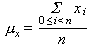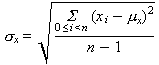On-line GuidesAll GuideseBook StoreiOS / AndroidLinux for BeginnersOffice ProductivityLinux InstallationLinux SecurityLinux UtilitiesLinux VirtualizationLinux KernelSystem/Network AdminProgrammingScripting LanguagesDevelopment ToolsWeb DevelopmentGUI Toolkits/DesktopDatabasesMail SystemsopenSolarisEclipse DocumentationTechotopia.comVirtuatopia.comHow To GuidesVirtualizationGeneral System AdminLinux SecurityLinux FilesystemsWeb ServersGraphics & DesktopPC HardwareWindowsProblem SolutionsPrivacy Policy## Tuple Exercises

These exercises implement some basic statistical algorithms. For some background on the Sigma operator, Σ, see the section called “Digression on The Sigma Operator”.

1. Blocks of Stock. A block of stock as a number of attributes, including a purchase date, a purchase price, a number of shares, and a ticker symbol. We can record these pieces of information in a `tuple` for each block of stock and do a number of simple operations on the blocks.

Let's dream that we have the following portfolio.

Purchase Date Purchase Price Shares Symbol Current Price
25 Jan 2001 43.50 25 CAT 92.45
25 Jan 2001 42.80 50 DD 51.19
25 Jan 2001 42.10 75 EK 34.87
25 Jan 2001 37.58 100 GM 37.58

We can represent each block of stock as a 5-tuple with purchase date, purchase price, shares, ticker symbol and current price.

```portfolio= [ ( "25-Jan-2001", 43.50, 25, 'CAT', 92.45 ),
( "25-Jan-2001", 42.80, 50, 'DD', 51.19 ),
( "25-Jan-2001", 42.10, 75, 'EK', 34.87 ),
( "25-Jan-2001", 37.58, 100, 'GM', 37.58 )
]
```

Develop a function that examines each block, multiplies shares by purchase price and determines the total purchase price of the portfolio.

Develop a second function that examines each block, multiplies shares by purchase price and shares by current price to determine the total amount gained or lost.

2. Mean. Computing the mean of a `list` of values is relatively simple. The mean is the sum of the values divided by the number of values in the `list`. Since the statistical formula is so closely related to the actual loop, we'll provide the formula, followed by an overview of the code.

Equation 13.1. MeanThe definition of the Σ mathematical operator leads us to the following method for computing the mean:

Procedure 13.1. Computing Mean

1. intialize sum, s , to zero

2. for each value, i , in the range 0 to the number of values in the `list`, n :

1. add element xi to s

3. return s divided by the number of elements, n

3. Standard Deviation. The standard deviation can be done a few ways, but we'll use the formula shown below. This computes a deviation measurement as the square of the difference between each sample and the mean. The sum of these measurements is then divided by the number of values times the number of degrees of freedom to get a standardized deviation measurement. Again, the formula summarizes the loop, so we'll show the formula followed by an overview of the code.

Equation 13.2. Standard DeviationThe definition of the Σ mathematical operator leads us to the following method for computing the standard deviation:

Procedure 13.2. Computing Standard Deviation

1. compute the mean, m

2. intialize sum, s , to zero

3. for each value, xi in the `list`:

1. compute the difference from the mean, d as xi - m

2. add d 2 to s . This is typically done as d * d in Java, since there is no “squared” operator. In Python, this can be d **2.

4. compute the variance as s divided by ( n - 1). This n - 1 value reflects the statistical notion of “degrees of freedom”, which is beyond the scope of this book.

5. return the square root of the variance.

The `math` module contains the `math.sqrt` funtion. For some additional information, see the section called “The `math` Module”.Published under the terms of the Open Publication License Design by Interspire